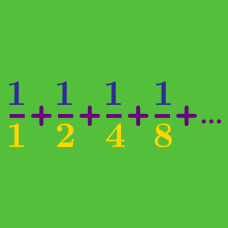Calculus

# Sequences Warmup

If $\{a_n\}$ is a sequence defined by $a_n = n^2 + n + 1$ for each natural number $n$, what is $a_5?$

Suppose $\{a_n\}$ is a sequence defined by $a_1 = 1, a_2 = 1,$ and $a_n = a_{n-1} + a_{n -2}$ for each natural number $n > 2.$

What is $a_6$?

A geometric progression is a sequence in which $a_n = r \cdot a_{n-1}$ for each natural number $n > 1$, where $r$ is a real number called the common ratio.

If $a_n$ is a geometric progression with $a_1 = 5$ and $a_6 = 160$, what is $a_3$?

On Day 1, Isabel has $200 in the bank, and she adds$5 at the start of each subsequent day. On what day will her account’s value reach $300? Each day this week, Morgan had twice as much money as the day before. On Day 6, Morgan had$40. How many dollars did Morgan have on Day 1?

×